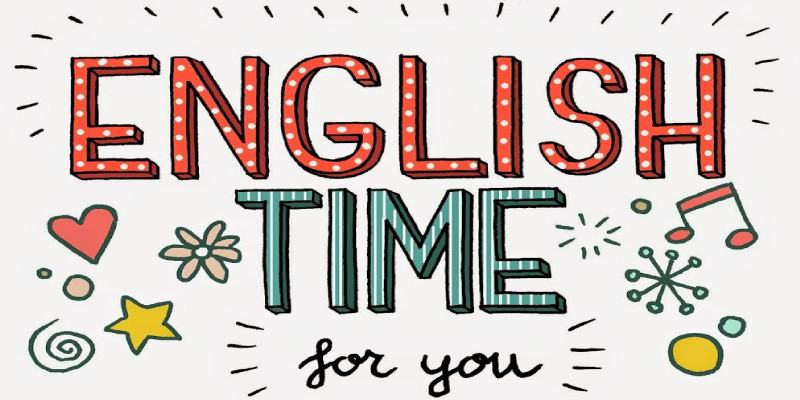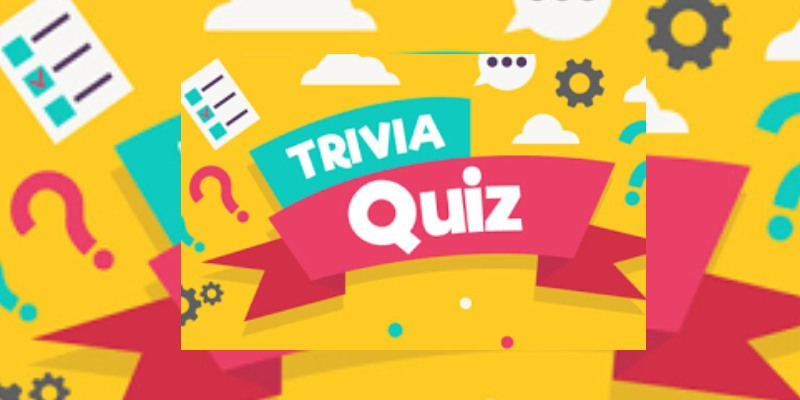### Mathematics Quiz Questions For 1st Grade Students#### 1. Find the addition of the two numbers,159+239=?

 97283 299 97284 398 97285 388 97286 293

#### 2. Find the subtraction of the two numbers, 769-539?

 97287 230 97288 236 97289 256 97290 259

#### 3. Find the subtraction of the three numbers. 29-12-6?

 97291 17 97292 14 97293 11 97294 21

#### 4. Find the multiple of the two numbers,13×16?

 97295 180 97296 206 97297 199 97298 208

#### 5. Find the addition of the three numbers,39+21+15=?

 97299 75 97300 79 97301 65 97302 71

#### 6. The multiple of 19×21 is ...

 97303 386 97304 367 97305 399 97306 349

#### 7. Find the subtraction of the two numbers,863-649=?

 97307 203 97308 195 97309 199 97310 214

#### 8. Find the addition of the three numbers,49+25+54=?

 97311 141 97312 119 97313 128 97314 111

#### 9. Find the multiple of the two numbers,21×33=?

 97315 693 97316 598 97317 649 97318 637

#### 10. The subtraction of 969-295 is...

 97319 649 97320 674 97321 599 97322 587

#### 11. The addition of 899+649 is ...

 97323 1577 97324 1457 97325 1548 97326 1518

### Let's Take More Quizzes

#### English Quiz For 1st Grade Students367399 Played 15-May-2020
This quiz is very interesting for 1st-grade students. In this quiz, you can learn about the day, month, and year. If you are 1st-grade students then y...

#### Mixed Trivia Quiz For 1st Grade365613 Played 07-May-2020
This quiz is meant for 1st grade, quiz help students identify what they know and what they don't know. These educational quizzes are designed to build...

View All